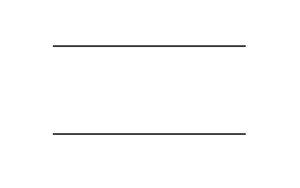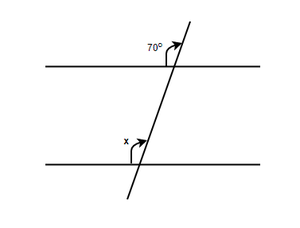# What are the properties of parallel lines?

• Last Updated : 21 Dec, 2021

The branch of math that deals with lines, angles, points, segments, etc is known as geometry. It shows the spatial relationship between different objects. The three major types of geometry are Euclidean geometry, Hyperbolic geometry, and Spherical geometry. Lines are a part of Euclidean geometry. Let’s start by learning what are lines and then moving on to parallel lines.

### Lines

It can be defined as the collection of points that extends infinitely. It was introduced by an ancient mathematician to determine straight objects having no thickness or depth. It doesn’t have any ends and is one-dimensional. Basically, there are four types of lines. They are mentioned below:

• Vertical lines: When a line moved from up to down in a straight direction it is called a vertical line.
• Horizontal lines: When a line moves from left to right in a straight direction it is called a horizontal line;
• Parallel lines: When two different lines moving in a straight direction doesn’t meet or intersect each other even in infinity they are called parallel lines.
• Perpendicular lines: When two lines meet or intersect each other at 90 degrees they are called perpendicular lines.

### Parallel lines

When two lines moving in a straight direction doesn’t meet or intersect each other even in infinity they are called parallel lines. Some of the real-life examples of parallel lines are railway tracks, edges of sidewalks, zebra crossing, railings, etc. The significance of parallel lines is not only seen in maths but also in real life.### What are the properties of parallel lines?

Following are the properties of parallel lines,

1. The pairs of vertically opposite angles are equal.
2. The pairs of corresponding angles are equal.
3. The pairs of alternate exterior angles are equal.
4. The pairs of alternate interior angles are equal.
5. The pair of interior angles lying on the same side of transversal is supplementary.

### Sample Questions

Question 1: What are the perpendicular lines?

Perpendicular lines are the lines that intersect each other exactly at a right angle or 90 degrees.

Question 2: What are the properties of a perpendicular line?

The properties of perpendicular line are:

1. Perpendicular lines always intersect each other.
2. Perpendicular lines move straight to the upwards direction from the intersecting point.
3. The angle between two perpendicular lines is always 90 degrees.

Question 3: What are the different types of lines?

The different types of lines we observe are:

• straight line
• curved line
• parallel line
• perpendicular line
• intersecting line

Question 4: Where do the parallel lines meet?

The parallel lines meet at the point of infinity.

Question 5: In the given figure, what is the value of the angle x?Solution:

According to the 2nd property of parallel lines, the corresponding angles are equal, Therefore,

x = 70°

My Personal Notes arrow_drop_up